## Step 1: Determine the least common denominator

If the denominators are not the same, determine the least common denominator (LCD) shared by the fractions. For example, let’s look for the denominator of the following: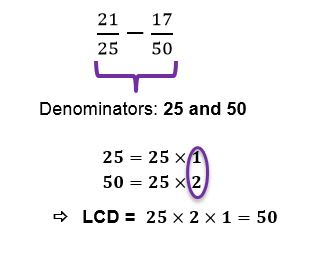## Step 2: Express the fractions to share the lowest common denominator

Express the fractions so that they share the LCD as their denominator. Hence, we express 21/25 and 17/50 so that they share 50 as the denominator.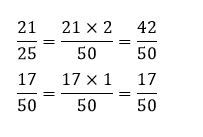## Step 3: Subtract the numerators

Now that the denominators are the same, we can subtract the numerators directly.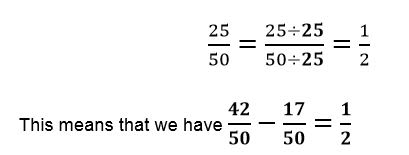## Step 4: Cancel the common factors

Simplify the difference of the fractions by cancelling out common factors.## Examples of how to subtract fractions

Q1) What is the result if we subtract 11/40 from 19/40?

Since they share the same denominators, we can subtract the numerators right away.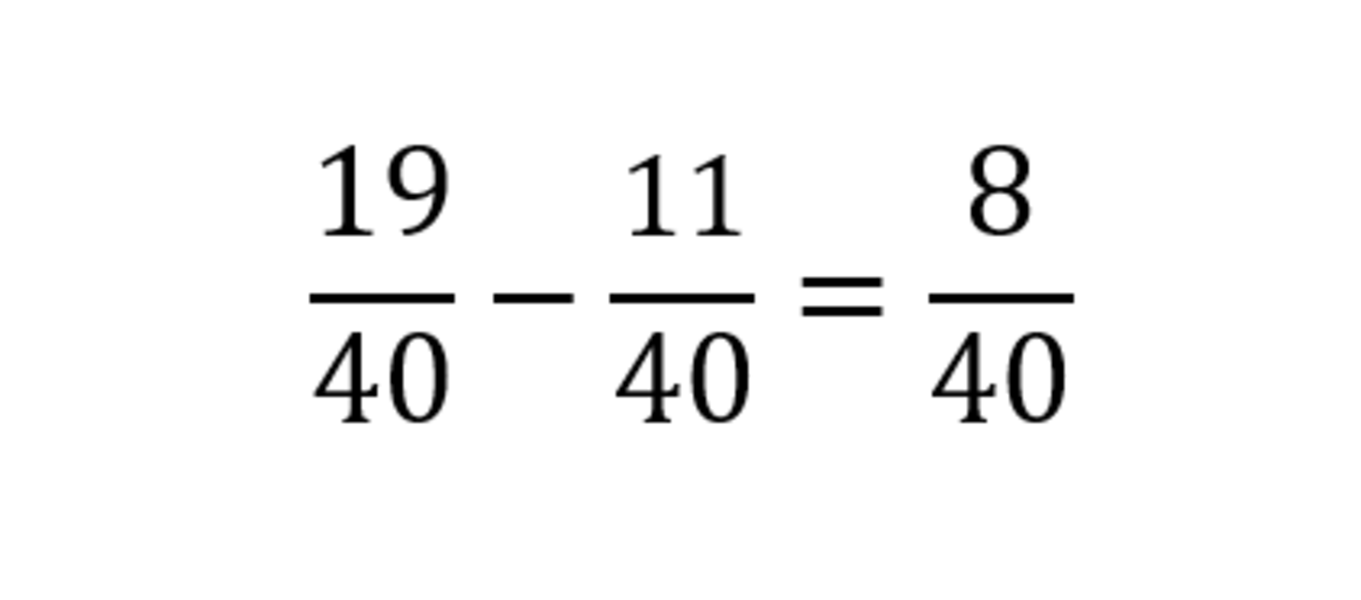Simplify the difference by cancelling out the common factors.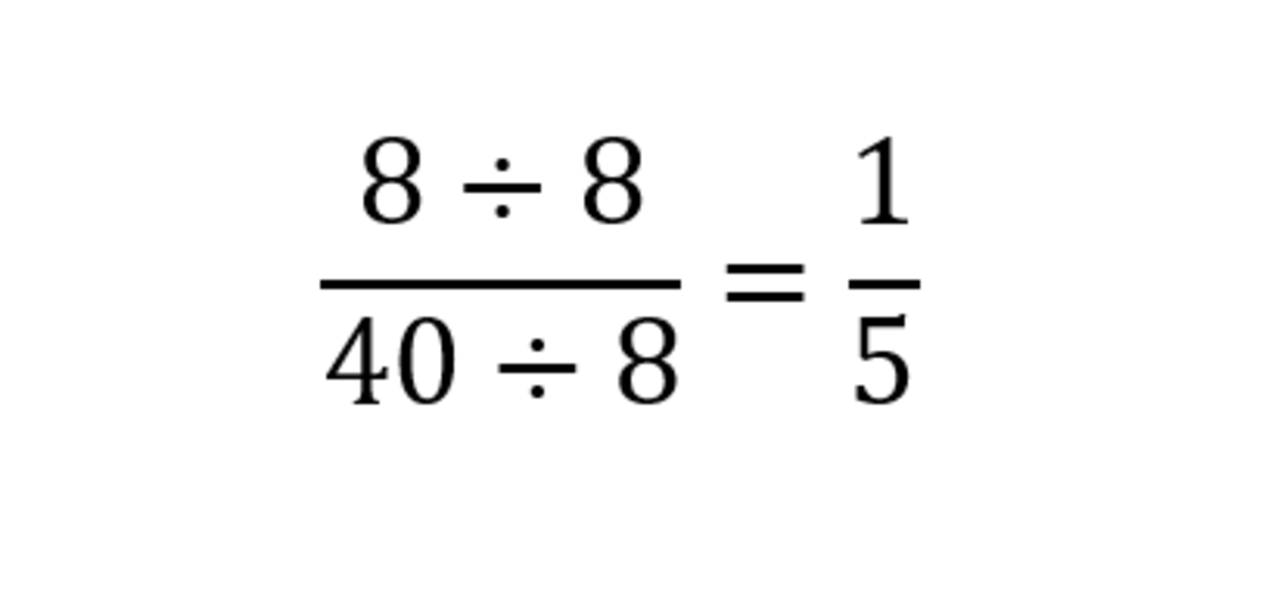Q2) Blake has ordered 4/5 tons of bananas for his banana chip business. His first batch of banana chips required 11/30 tons of bananas. How much of the bananas are left?

To find the remaining amount of bananas, we subtract 11/30 from 4/5.

Since the denominators are not the same, we’ll need to find the LCD shared by the fractions.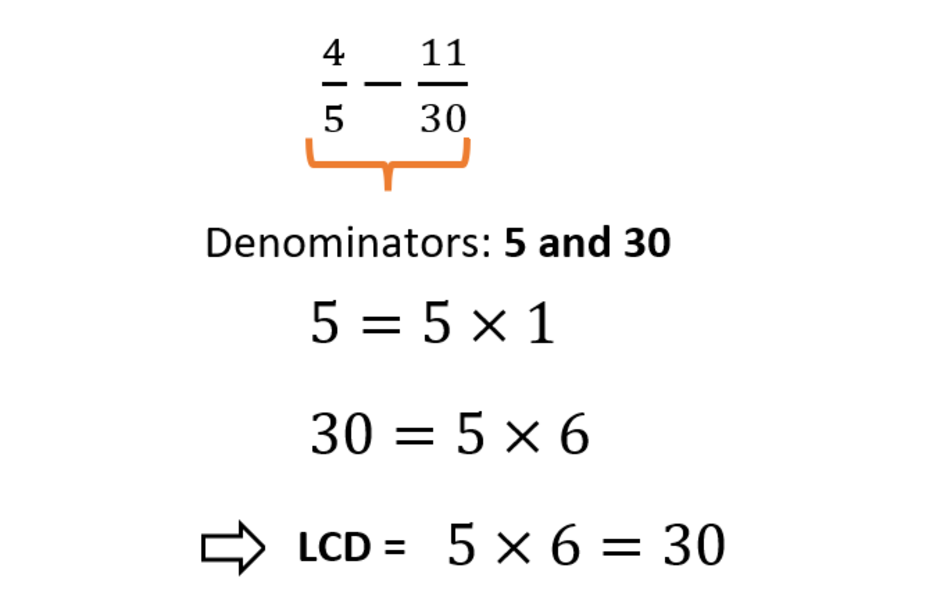Now, rewrite the fractions so that they both have 30 as their denominator.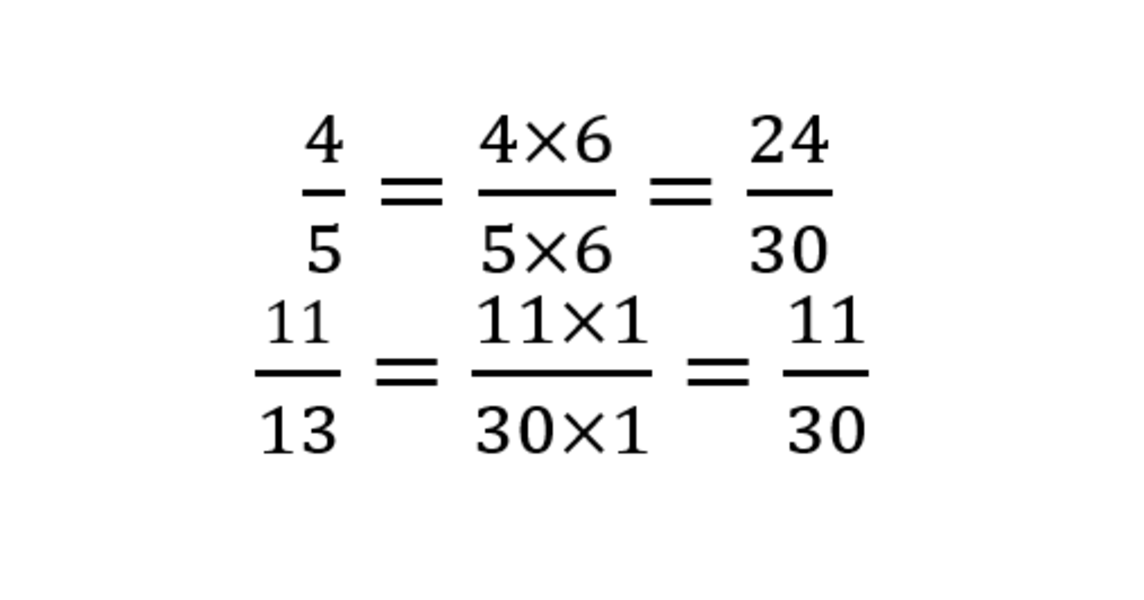Once the fractions share a common denominator, we can now subtract the numerators right away.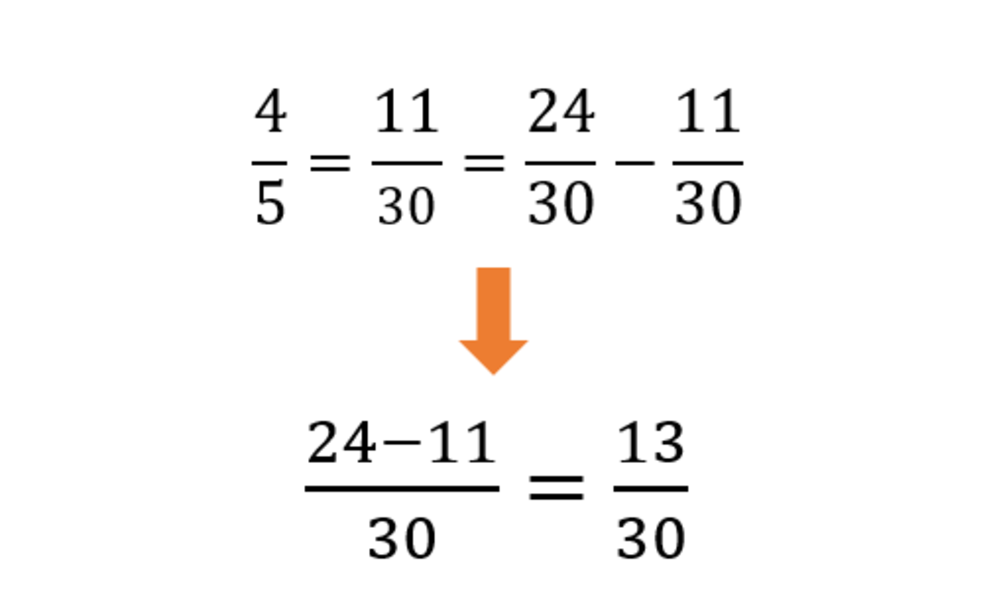This means that Blake would still have 13/30 tons of bananas left after the first batch.